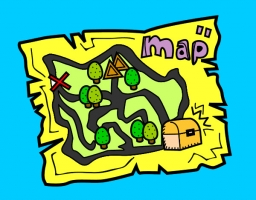# Calculate 55981

Calculate the following example:
On a map with a scale of 1:50,000, two places, A and B, are 4 cm apart. Find out:
a) what is the distance between these places in reality;
b) what is the scale of the map in which these places are 12 cm apart?

x =  2 km
M2 =  3:50000

### Step-by-step explanation:Did you find an error or inaccuracy? Feel free to write us. Thank you!

Tips for related online calculators
Do you want to convert length units?